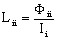# Basic definitions: magnetic flux, inductance,...

## Magnetic flux: definition

Let there be a filiform circuit closed-loop, defined by its contour C and provided with an electric current I.

The magnetic flux Φ associated with the contour C is defined by: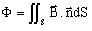(1)

where:

•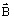is the magnetic field created by the current I
•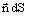is the vector element of surface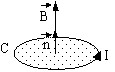Stokes' theorem expresses the magnetic flux as a line integral, in the following form: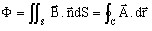where:

•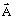is the magnetic vector potential (such as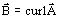)
•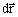is the vector element of line

## Inductance: definition

The inductance of a filiform circuit closed-loop, defined by its contour C and provided with an electric current I, is defined by the formula: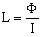It can be expressed by one of the two following formulas: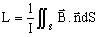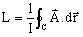## Self-inductance and mutual inductance

Let i and j be two filiform circuit closed-loops, defined by their contour Ci and Cj and flowed through by the electric currents Ii and Ij.

The mutual inductance between i and j circuits is defined by the formula: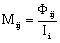where Φij is the magnetic flux through the closed-loop Cj generated by the magnetic field produced exclusively by the current Ii.

With respect to this last definition, the self-inductance of the closed-loop Ci corresponds to the particular case where i = j: